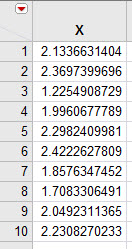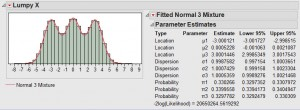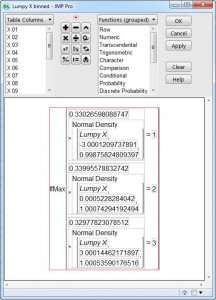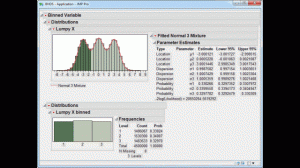Our World Statistics Day conversations have been a great reminder of how much statistics can inform our lives. Do you have an example of how statistics has made a difference in your life? Share your story with the Community!
Choose Language Hide Translation Bar

There is usually a desire to have the most precise measurement of any measurement. In theory, that is good, but for the purposes of data analysis, more precision isn't always better.

It is usually best to examine any continuous variable and determine a reasonable precision for the recorded values. For instance, suppose I have a variable X, and X has the values shown in the table below:The data is recorded to 10 decimal places. But if we are analyzing this data, do we really need that many digits to the right of the decimal? One way to examine this is to look at the plot of the estimates of the mean and standard deviation of this data, as a function of the level of rounding used. A good question to ask is "How sensitive are the summary statistics to the level of precision?"

A rule of thumb

The table and the chart show that we don't lose very much information in the estimate of the mean even if we round all the way to one decimal place. A good rule of thumb about the needed precision for a variable is to divide the standard deviation of the unrounded data by 3, and use the leading significant digits decimal place as the level of rounding. In the example shown here, the standard deviation of the unrounded data is 0.3612, so 1/3 of that is 0.12, indicating that one decimal place is sufficient.

Does this matter when building models?

Recently, I encountered a predictive modeling problem where the time it took to fit a decision tree model was longer than desired (many hours of computational time). The data set being used was fairly large (several million rows). Investigating the problem, I discovered that many of the continuous predictor variables (the Xs, or the factors) were recorded out to many decimal places. Using the rounding rule of thumb, the computation time for fitting a decision tree model decreased to several minutes. Why did this happen? The recursive partitioning algorithm that builds decision trees uses the unique levels of each continuous predictor and builds binary splits based on each unique level in order to find the optimum split. If the factors in the model are overly precise, this adds a large amount of computational overhead with often very little benefit in improved model accuracy. Rounding the factors reduced the number of unique levels and made the model fitting algorithm perform much faster.

Binning Data

In practice, it is also known that even less precise indicators of the levels of continuous variables can be useful and lead to better models. One approach to reducing the precision of a predictor variable is to employ binning. Binning simply assigns each continuous variable to a categorical level. Rounding is one example of binning. Another example of binning is to build a histogram of the data and using the bins in the histogram as the predictor. A previous blog post described an interactive binning tool that you can use to do this sort of binning manually. A third example of binning is to use a supervised approach, where the bins in the predictor are chosen in a way that maximizes the predictive ability of the binned variable.

For the data analysis problem I faced, with overly precise data leading to long computational time, I had 100 predictor variables that needed some level of binning applied. The interactive binning approach would have taken too long, and the supervised binning approach is, in itself, computationally intense, so it was taking quite a bit of time. I decided to employ an unsupervised binning approach that simply looks for groupings or clusters in the predictor variables, one variable at a time.

Binning Data Using Normal Mixture Distributions

Consider the slightly "lumpy" variable shown in the histogram below. A Normal Mixture distribution using 3 normal distributions is fit to the data (Hotspot>Continuous Fit>Normal Mixtures>Normal 3 Mixture). The parameter estimates from the mixture distribution are recorded, and a binning formula is created that assigns a row to a group based on which distribution in the mixture the row has the highest associated probability.The binned variable is an integer that preserves the ordering in the data, so it can be used as a continuous, ordinal or nominal variable.Click image to see animated version

To automate the process of using normal mixture distributions for unsupervised binning, I created a JMP add-in (available for download on the JMP File Exchange -- requires a free SAS profile).

As part of your data preparation for modeling, take into account the data precision of your predictor variables, and see if lower precision might be helpful as you do your analysis.  I hope the ideas shared in this post are useful to you as you work to build better models.

Article Labels

There are no labels assigned to this post.

Article Tags
Visitor

chris dorger wrote:

Sam,

interesting rule of thumb and easy to remember. Do you have a reference for its source? Why does it work?

Visitor

Sam Gardner wrote:

One reference that mentions the usefulness of binning is Berry & Linhoff, Data Mining Techniques, 2nd Ed, p 237 and p351. In particular, they state "Perhaps unexpectedly, binning values can improve the performance of data mining algorithms."

Visitor

Sam Gardner wrote:

the link to the script is no longer active. The new link to the binning script is at https://community.jmp.com/docs/DOC-7584Staff

Arati Mejdal wrote:

Thanks, Sam! I will update the link in the post.